Warning

This documents an unmaintained version of NetworkX. Please upgrade to a maintained version and see the current NetworkX documentation.

biadjacency_matrix(G, row_order, column_order=None, dtype=None, weight='weight', format='csr')[source]

Return the biadjacency matrix of the bipartite graph G.

Let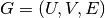be a bipartite graph with node sets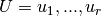and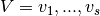. The biadjacency matrix  is the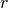x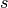matrix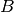in which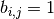if, and only if,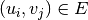. If the parameter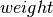is not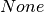and matches the name of an edge attribute, its value is used instead of 1.

Parameters: G (graph) – A NetworkX graph row_order (list of nodes) – The rows of the matrix are ordered according to the list of nodes. column_order (list, optional) – The columns of the matrix are ordered according to the list of nodes. If column_order is None, then the ordering of columns is arbitrary. dtype (NumPy data-type, optional) – A valid NumPy dtype used to initialize the array. If None, then the NumPy default is used. weight (string or None, optional (default=’weight’)) – The edge data key used to provide each value in the matrix. If None, then each edge has weight 1. format (str in {‘bsr’, ‘csr’, ‘csc’, ‘coo’, ‘lil’, ‘dia’, ‘dok’}) – The type of the matrix to be returned (default ‘csr’). For some algorithms different implementations of sparse matrices can perform better. See  for details. M – Biadjacency matrix representation of the bipartite graph G. SciPy sparse matrix

Notes

No attempt is made to check that the input graph is bipartite.

For directed bipartite graphs only successors are considered as neighbors. To obtain an adjacency matrix with ones (or weight values) for both predecessors and successors you have to generate two biadjacency matrices where the rows of one of them are the columns of the other, and then add one to the transpose of the other.

adjacency_matrix(), from_biadjacency_matrix()